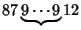## Reversal

The reversal of a decimal numberis. Ball and Coxeter (1987) consider numbers whose reversals are integral multiples of themselves. Palindromic Numbers and numbers ending with a Zero are trivial examples.

The first few nontrivial examples are 8712, 9801, 87912, 98901, 879912, 989901, 8799912, 9899901, 87128712, 87999912, 98019801, 98999901, ... (Sloane's A031877). The pattern continues for large numbers, with numbers of the formequal to 4 times their reversals and numbers of the formequal to 9 times their reversals. In addition, runs of numbers of either of these forms can be concatenated to yield numbers of the form, equal to 4 times their reversals, and, equal to 9 times their reversals.

The product of a 2-digit number and its reversal is never a Square Number except when the digits are the same (Ogilvy 1988). Numbers whose product is the reversal of the products of their reversals include(Ball and Coxeter 1987, p. 14).

References

Ball, W. W. R. and Coxeter, H. S. M. Mathematical Recreations and Essays, 13th ed. New York: Dover, pp. 14-15, 1987.

Ogilvy, C. S. and Anderson, J. T. Excursions in Number Theory. New York: Dover, pp. 88-89, 1988.

Sloane, N. J. A. Sequence A031877 in The On-Line Version of the Encyclopedia of Integer Sequences.'' http://www.research.att.com/~njas/sequences/eisonline.html.